# Flood Forecasting using Machine Learning Models

India has diverse cultures and unpredictable weather, especially the monsoon. According to the International disaster dataset EM-DAT, over 1200 people die annually in India due to floods. India loses more than \$7 billion yearly because of natural disasters, including \$3 billion alone from floods. A sound alerting system and accurate machine learning model can help prevent all these deaths and damage to the infrastructure.

Google has already taken the initiative to help countries by making its AI-based flood prediction model to predict flood-prone areas near the rivers so they can be evacuated quickly. Our blog will focus on considering rainfall amounts to understand the pattern of floods across India and make a machine-learning model to predict it.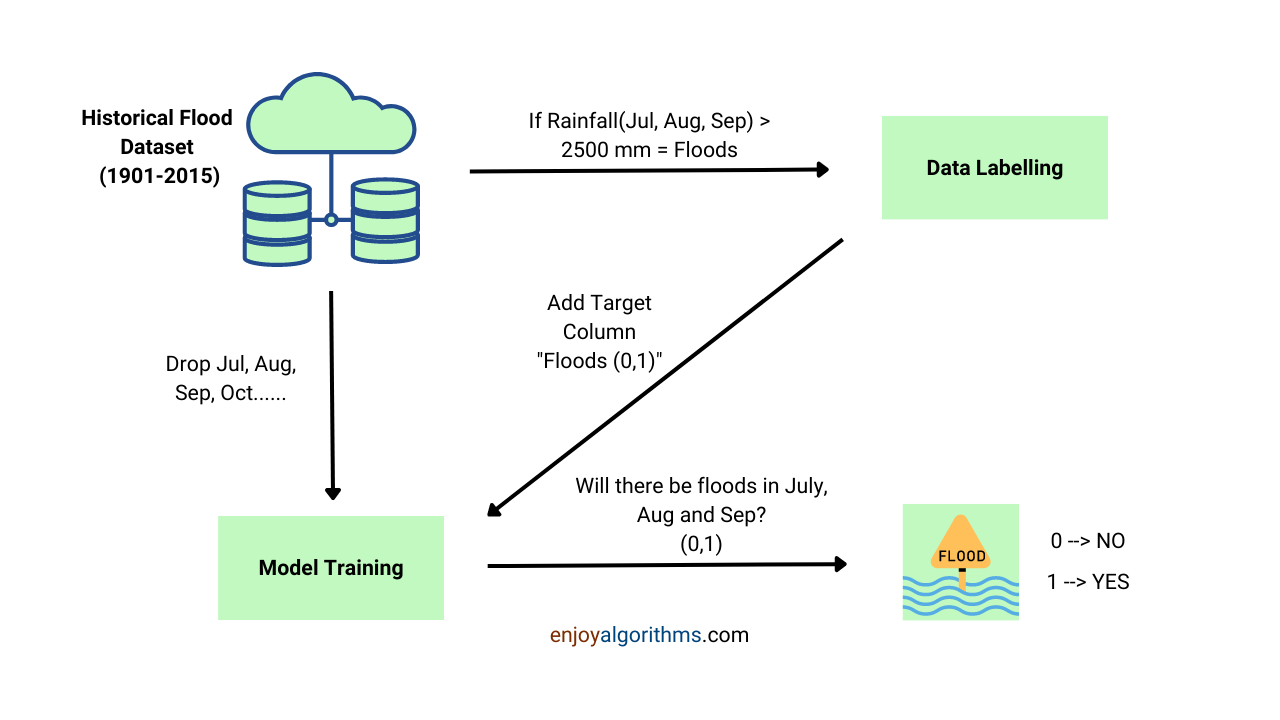### Key points

1. The Kerala story
3. Comparison in Machine Learning and Artificial Neural Network Models

Kerala, Himachal Pradesh, West Bengal, and Orissa are some of the most affected states by floods in India. These four states comprise more than 70% of deaths caused by floods every year. To save the life of these people, we have to connect with their problems and the factors triggering these disasters to choose the proper parameters for our model.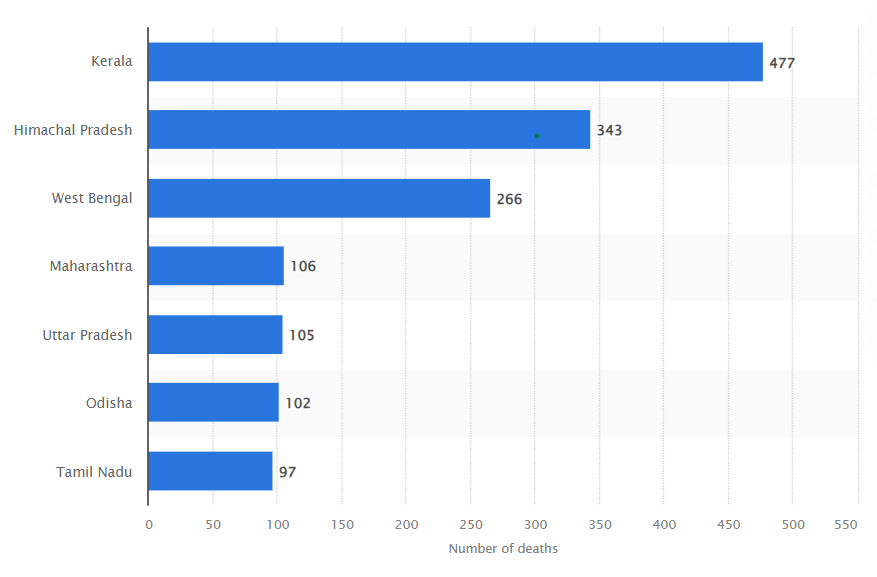### Problem Statement

We have the data on the monthly rainfall of all the Indian states from 1901 to 2015. We aim to study each state and understand its topology, rainfall patterns, and most prominent monsoon months (e.g. Jul, Aug, Sep). Then find out the average precipitation in these months and label the years having rainfall more than this mark as flood-risk years. Then apply a machine learning model to predict the possibility of floods at the start of these months. We will not have the rainfall data for these months while training and testing our model. This step is only for data annotation. Let's start the research.

### The Kerala Story

Kerala has 39 major dams on more than 30 rivers. Continuous rainfall and the risk of overflow compels the officials to open the gates of these dams leading to an immense amount of water discharge submerging the low-lying areas. Here are some parameters that will help us understand the state's topography.

Major Flood causing rivers: Periyar, Meenachil, Pampa, Muvattupuzha, Manimala

Average Annual precipitation: 3055 mm

Monsoon months: May, June, July and August

Floods so far: 1924, 1961, 2018

Our dataset contains the monthly average rainfall of all the states of India from 1901 to 2015. One can download the dataset from Kaggle. Looking at the parameters affecting floods in Kerala, we concluded that May, June, July and August are more correlated and should be given more weightage. Therefore we will add one column containing the average rainfall of these four months. If these months cross their combined average of 2236 mm in precipitation, the chances of floods are high. The below graph also supports our findings.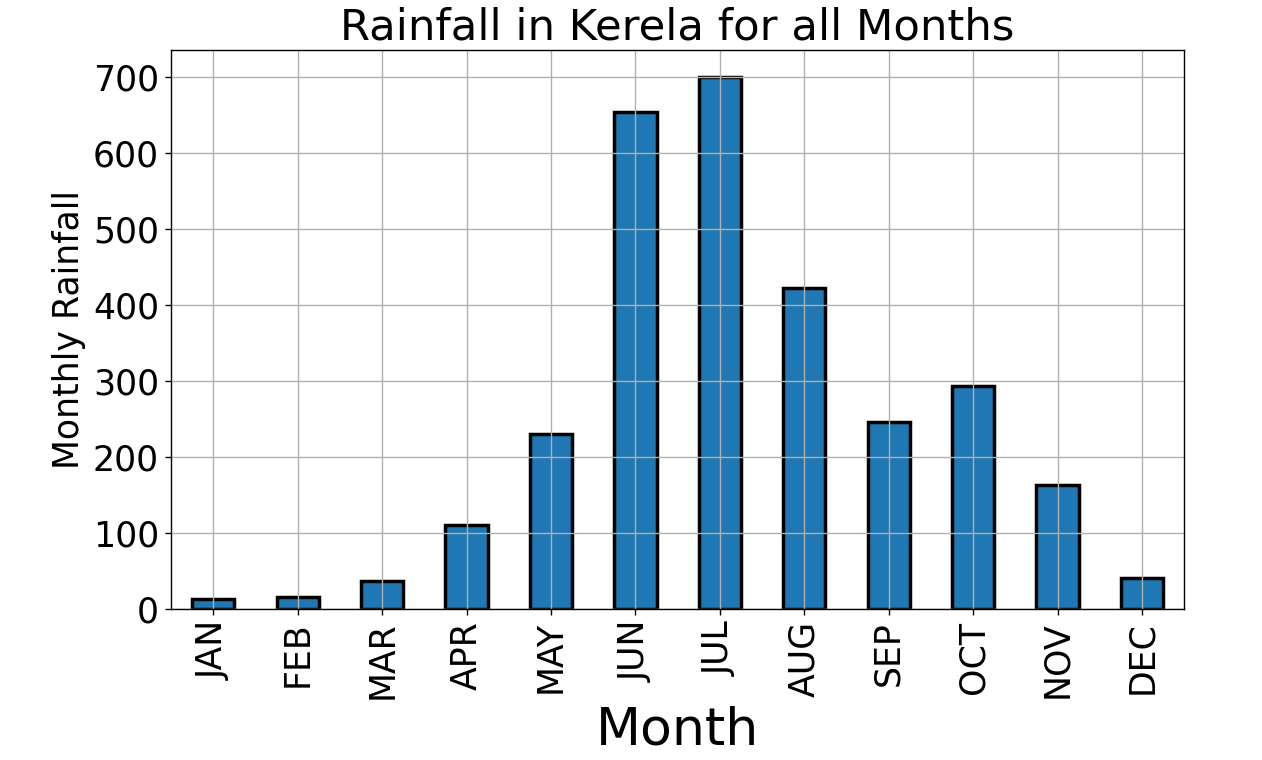We will consider rainfall from January to June's first week and predict whether the upcoming months will face floods. We will make Machine learning and Artificial Neural Network models and compare their performance on various evaluation metrics.

#### Dataset Description

The dataset contains 19 columns and 4090 rows after dropping null values. Of these 19 columns, 12 are for the months, and others are the cumulative sum of rainfalls.

SUBDIVISION, YEAR, JAN, FEB, MAR, APR, MAY, JUN, JUL, AUG, SEP, OCT, NOV, DEC, ANNUAL, Jan-Feb, Mar-May Jun-Sep, Oct-Dec.

Although we know that rainfall alone is not sufficient to predict floods, we tried to deliver the best result from what we had. We recommend you search other available weather datasets of Indian states to make a more robust model.

#### Data annotation

To label the data for floods, we took the average of the column "summed_rainfall" as a threshold. Years having rainfall above this average are marked as floods.

``````def data_maker(state_data, col_to_drop, col_to_sum):
state_data=state_data.drop("SUBDIVISION",axis=1)
sum_of_month=[]
state_data["summed_rainfall"] =state_data[col_to_sum]
for col in col_to_sum:
state_data["summed_rainfall"]+=state_data[col]

flood=[]
ann_prec=state_data["summed_rainfall"].mean()
for item in state_data["summed_rainfall"]:
if item>=ann_prec:
flood.append(1)
count+=1
else:
flood.append(0)

state_data["flood"]=flood

state_data=state_data.drop(col_to_drop,axis=1)

return state_data``````

We have to drop those month and annual rainfall columns to predict the possibility of floods in the months preceding June because their presence will make the model overfit.

#### Model building and evaluation

We should consider every possibility of improvement in applications involving high life risks. Seeing this, we implemented three machine learning models using different classification algorithms and also compared their performance with the artificial neural network.

``````models = []

from sklearn.svm import SVC
from sklearn.linear_model import LogisticRegression
from sklearn.ensemble import RandomForestClassifier

models.append(('LR', LogisticRegression()))
models.append(('SVC', SVC()))
models.append(('RF', RandomForestClassifier()))

names = []
scores = []
F1_Scores=[]
Recall=[]
for name, model in models:
model.fit(x_train, y_train)
y_pred = model.predict(x_test)
scores.append(accuracy_score(y_test, y_pred)*100)
F1_Scores.append(f1_score(y_test,y_pred)*100)
Recall.append((roc_auc_score(y_test,y_pred)*100))
names.append(name)
acc = pd.DataFrame({'Name': names, 'Accuracy Score': scores})
fsc = pd.DataFrame({'Name': names, 'F1 Score': F1_Scores})
rcl = pd.DataFrame({'Name': names, 'Recall Score': Recall})``````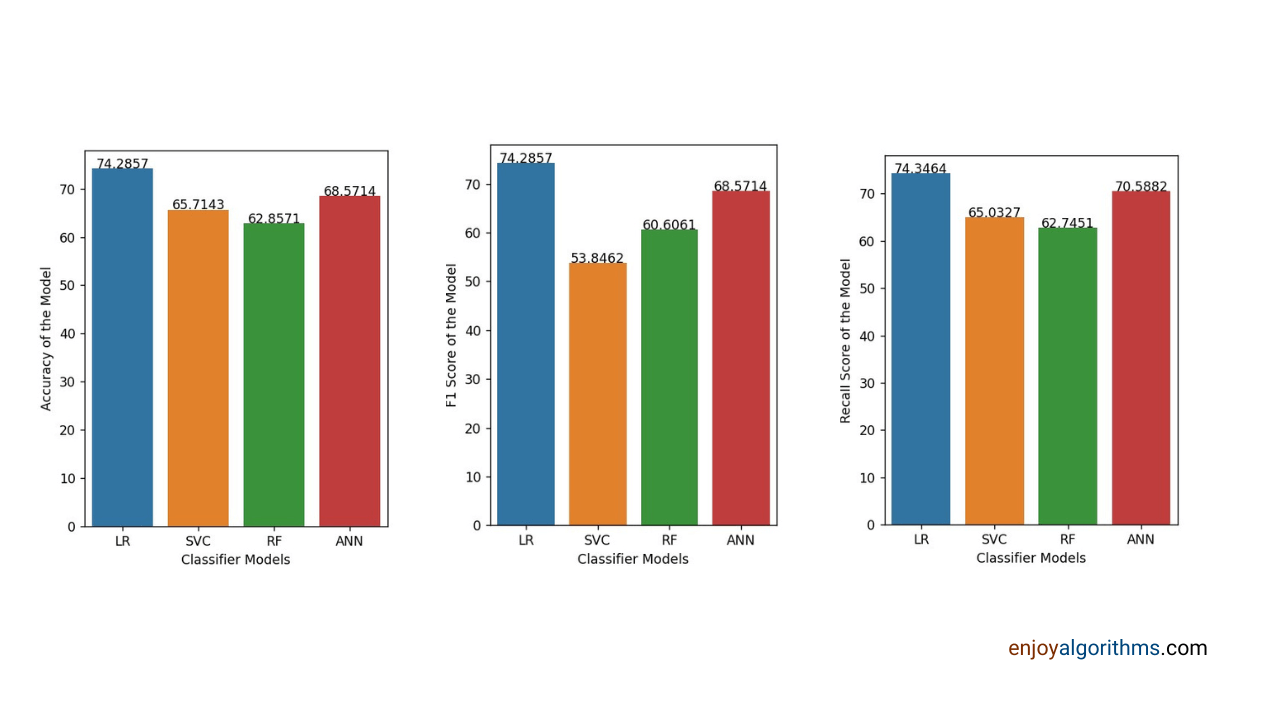The most important evaluation metric in this application is the recall score. Because if the model predicts no floods in case of floods, then the officials might have little time to prepare and evacuate the fields. Therefore we want our model to penalize false negative cases and thus need a high recall score. We can see that logistic regression has the highest recall score and performs better than neural networks and other machine learning algorithms. So now, let's implement the same methods on other states' data and see how the model performs.

Monsoon brings joy and sorrow at the same time in Himachal Pradesh. Floods occur every ten years here. Diverse topography, rainfall in mid and lower Himalayan ranges, blockage in Satluj and Beas basins, water flowing from steep slopes, glacier melts, cloud bursts, loosening of sand due to deforestation, and construction on river banks are responsible for massive floods in Himachal Pradesh.

Major Flood causing rivers: Satluj, Beas, Ravi, Yamuna

Average Annual precipitation: 1250 mm

Monsoon months: July, August and September

Floods so far: 1910, 1917, 1922–24, 1945, 1988, 1995, 2005, 2007, 2019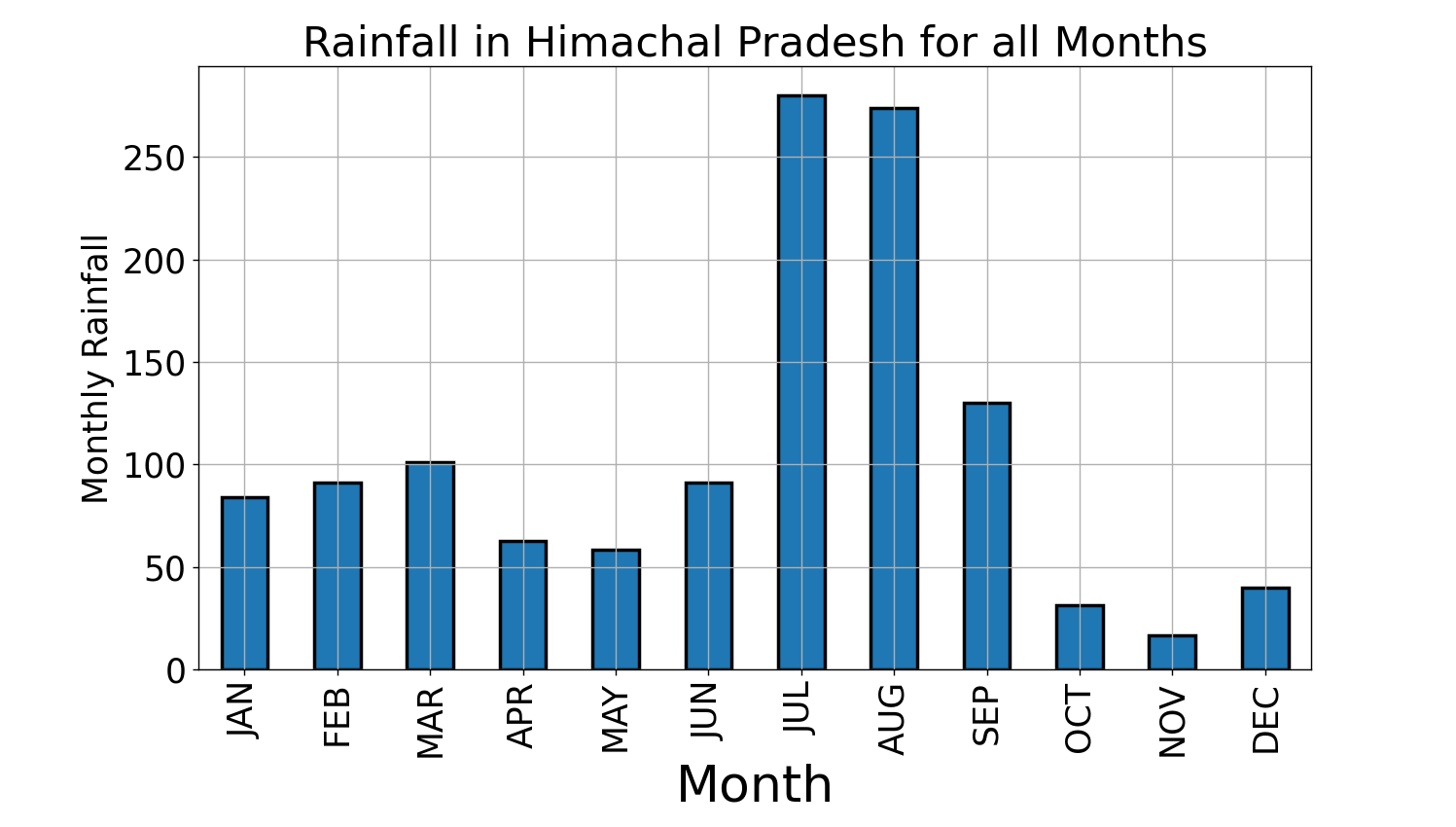There are three types of floods, among which two are more common in Himachal Pradesh.

1. Flash Floods: These floods result from heavy rainfall within a small duration of 5–10 hours. Especially when the slope is steep, and the path of flow is blocked, the water gets stored in the catchment area, and cloud bursts and tropical storms lead to the release of this immense amount of water.
2. River Floods: These floods build up slowly in comparison to flash floods. These are caused by consistent rainfall and water deposition from glacier melt. Due to increased riverbank construction, the land loosens and cannot hold much water.

We can start with the model building now with all the domain knowledge. Rainfall above average in April, May, and June results in water accumulation in catchment areas. The heavy rain of July, complemented by cloud bursts, led to flash floods. We will take the average of the combined rainfall of July, August, and September. If the rain crosses that average, the risk of floods increases. Our goal will be to consider rainfall till July's first week and then predict the possibility of floods.

#### Data Labelling and Processing

We have a dataset of all the states, so firstly, we segregated the data of Himachal Pradesh and then took the average of combined rainfall over 115 years. We took this average as a benchmark and put a check if HPdata["combinedrainfall"]>annprec, then marked it as a possible flood situation. The code for this data-making is already given in the Kerala story section. We have to pass statedata=HPdata, coltodrop and colto_sum.

``````def data_extractor(state):
state_data=data[data["SUBDIVISION"]==state ]
return state_data

HP_data=data_maker(HP_data,["YEAR","OCT","NOV","DEC"],["JUL","AUG","SEP"])``````

After calculating the ann_prec and labelling the data, we dropped these columns so they could not contribute to the model learning. Because in real-time, we will not have the data for July and months after it.

``````HP_data["JUL"]=HP_data["JUL"]/3
HP_data=HP_data.drop(["AUG","SEP","Mar-May", "Jun-Sep","Oct-Dec","Jan-Feb","ANNUAL", 'sumed_rainfall','flood'],axis=1)``````

It's time to build the Machine Learning and Artificial neural network model and evaluate their performance.

#### Model Building and Evaluation

As already mentioned, we used Logistic Regression, Support Vector Machine, Random Forest and ANN for this application, and the model with the best accuracy, recall and F-1 score will win. Let's see the configuration of the ANN model we used.

``````from sklearn.metrics import accuracy_score,recall_score,roc_auc_score,confusion_matrix,f1_score
import tensorflow as tf
model = tf.keras.models.Sequential()

model.fit(x_train,y_train,epochs=400,)
loss,accuracy=model.evaluate(x_test,y_test)``````

There is always a scope for improvement in machine learning. You can train the ANN model with a different set of hyperparameters to increase the model's performance. Some of these hyperparameters are the number of layers, the number of neurons per layer, and the number of epochs to train.

Now let's see the performance of these models!

``````def plot_show(data_to_plot, score_name, y_label):
axis = sns.barplot(x = 'Name', y = score_name, data =data_to_plot)
axis.set(xlabel='Classifier Models', ylabel=y_label)
for p in axis.patches:
height = p.get_height()
axis.text(p.get_x() + p.get_width()/2, height + 0.01, '{:1.4f}'.format(height), ha="center")

plt.show()``````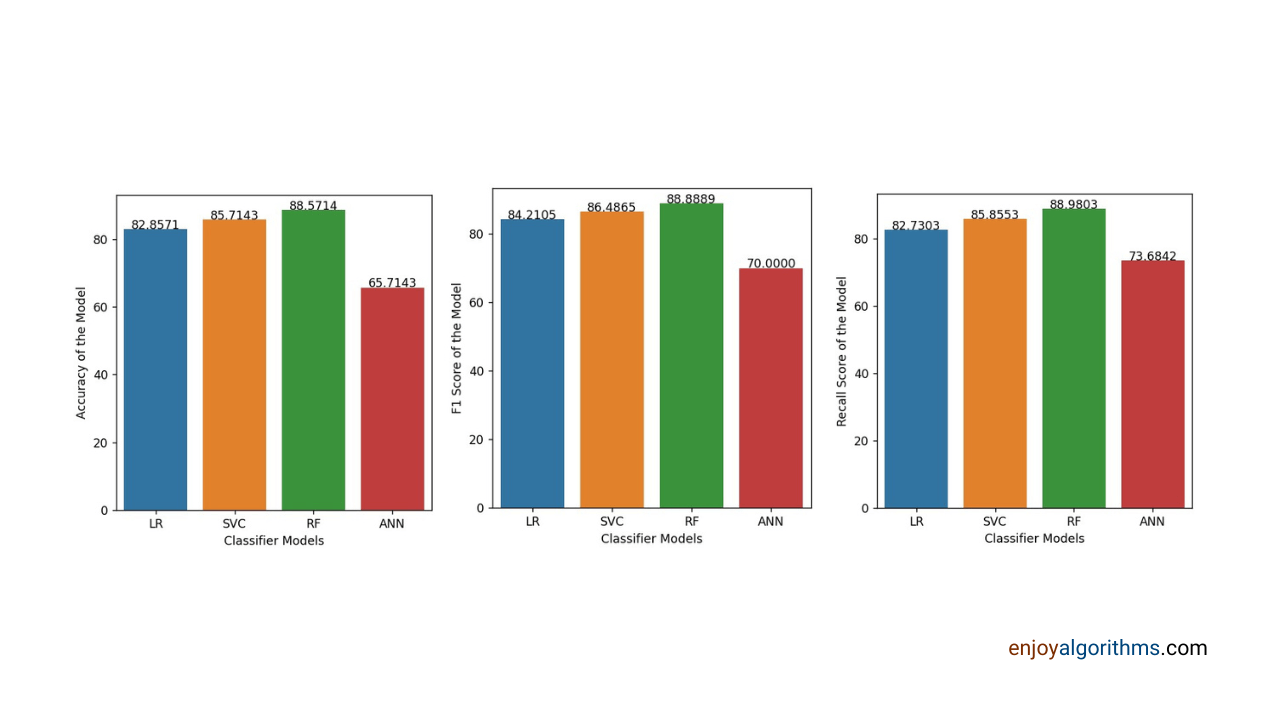The Kerala story section gives the code to find these evaluation metrics. Check it out from there. As we can see from the above graph, Random Forest Algorithm has performed better than the others. You might be wondering why the Neural Networks model is not performing well. The reason is the small dataset. After filtering out the dataset for a particular state, we get 115 rows which is very small for a neural network model to learn the patterns in the dataset. In contrast, tree algorithms work better with less data. Therefore random forest performed best among other ML algorithms.

We leave it to you to implement this code for other states and share the results in the feedback/review section of the blog.

### Case study

Google uses two models to predict when and where the floods will affect most. The Hydrologic model predicts the water level of the rivers using rainfall, weather and other basin-related data. The Inundation model uses satellite images to indicate the places near rivers which might expect floods. The forecast is accessible to everyone and is updated every day. The best thing is we can see the forecast results quickly, like google maps. We hope they reach every country and save more lives.

### Conclusion

Rainfall, storms, landslides, cyclones, floods and all other natural disasters are unpredictable. The best we can do is use modern technologies to forecast them and prepare beforehand. This blog aimed to convey a data scientist's thought process while making a machine learning model. First, we studied the state's history and got the benchmarks and months responsible for floods. Then we implemented three different ML algorithms and ANN to get the best results. Then we understood which evaluation metric is best to use in such applications.

☆ 16-Week Live DSA Course
☆ 10-Week Live DSA Course